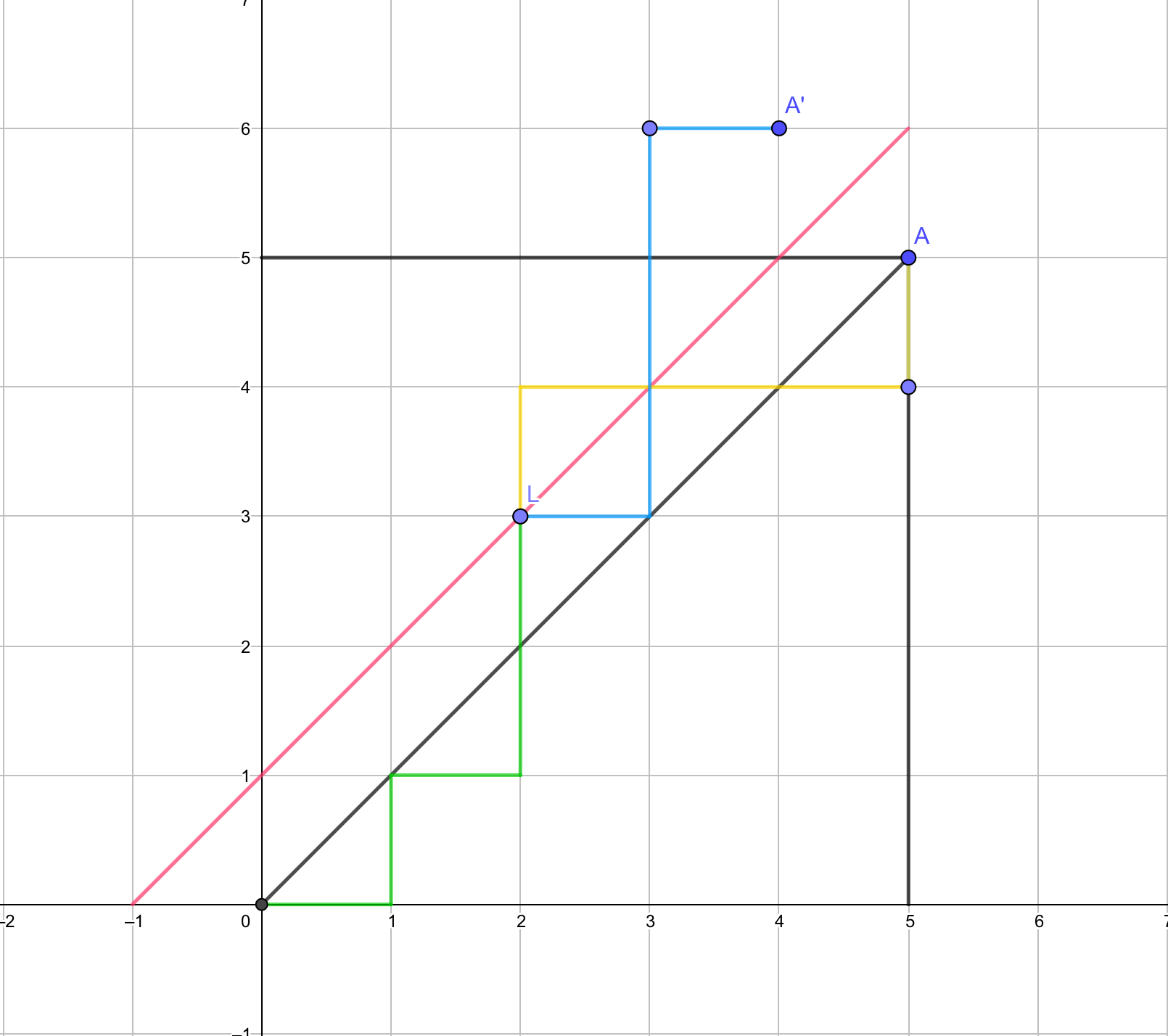# 卡特兰数 pufer序列 bsgs

## 卡特兰数

1, 1, 2, 5, 14, 42, 132, 429, 1430, 4862, 16796, 58786, 208012, 742900, 2674440......

\(h(n)\)\(catalan\)数的第\(n\)

\(h(0)=1\),\(h(1)=1\)

\(h(n)= h(0)*h(n-1)+h(1)*h(n-2) + ... + h(n-1)*h(0) (n≥2)\)

\(h(n)=h(n-1)*(4*n-2)/(n+1)\)

\(h(n)=C(2n,n)/(n+1)\).................(1)

\(h(n)=C(2n,n) - C(2n,n-1)\)..................(2)

\(C_n\)表示所有在\(n×n\)格点中不越过对角线的单调路径的个数（只向上向右走）\(C_{2n}^n-c_{2n}^{n-1}\)

### 网格

\(C_{n+m}^n-C_{n+m}^{n+1}\)

\((n+m)!*(n-m+1)/(n+1)!m!\)

## pufer序列

pufer序列与无根树存在对应关系

\(n\)个点的无根树得到的pufer序列长度为\(n-2\)

• 找到编号最小的叶节点，将他的父亲加入pufer序列，删除该节点

• 重复操作至剩余两个节点

pufer序列转无根树

• 取出prufer序列最前面的元素x

• 取出在点集中的、且当前不在prufer序列中的最小元素y

• 在x,y之间连接一条边

• 最后在点集中剩下的两个点中连一条边

1. pufer序列与无根树一一对应

2. 度数为k的点在pufer序列中出现k-1次

3. 一个n个点的完全图的生成树的个数为\(n^{n-2}\)

THE END